# Dividend policy, growth, and the valuation of shares

Oct. 12, 2012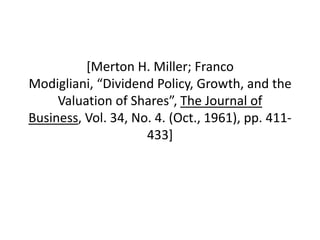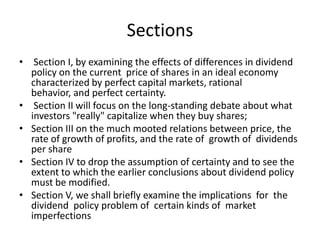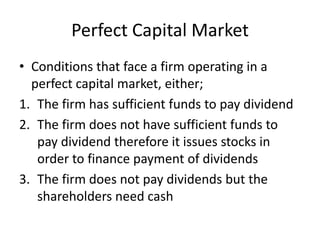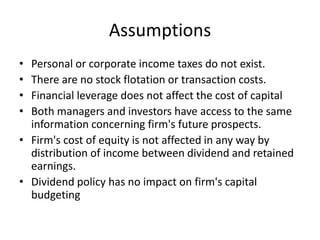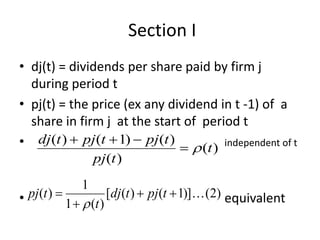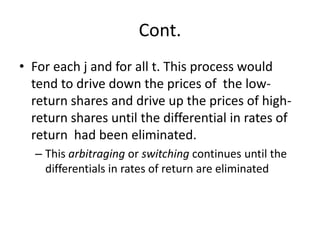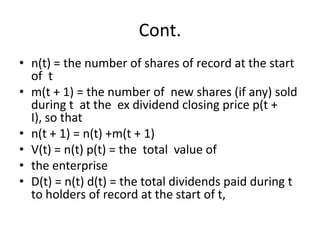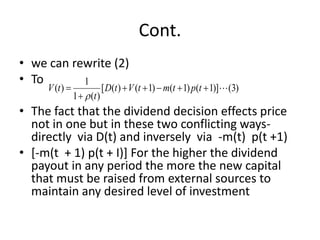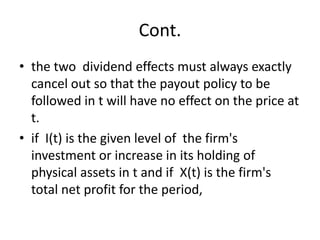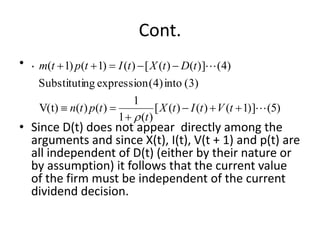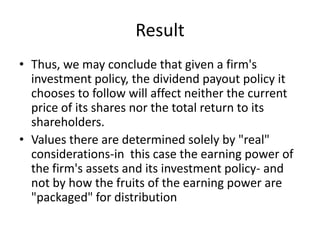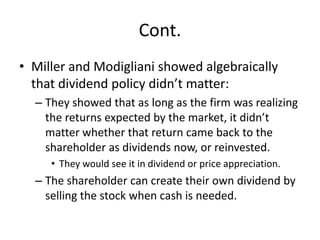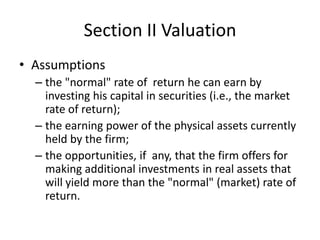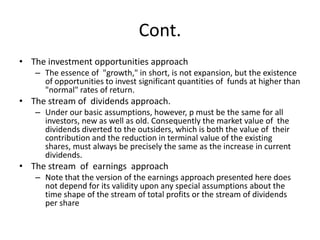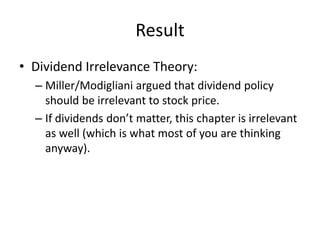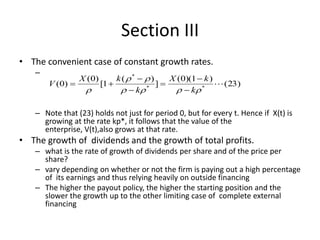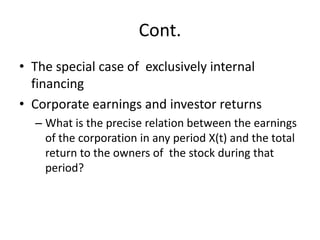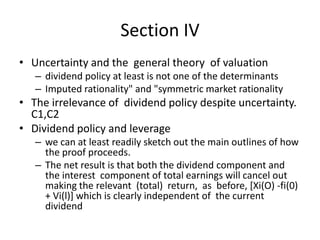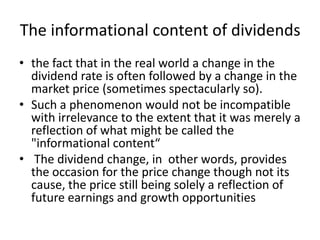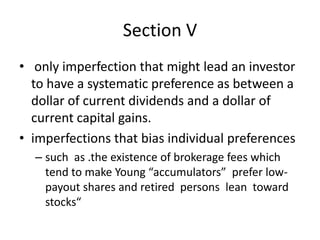1 of 21

### Dividend policy, growth, and the valuation of shares

1. [Merton H. Miller; Franco Modigliani, “Dividend Policy, Growth, and the Valuation of Shares”, The Journal of Business, Vol. 34, No. 4. (Oct., 1961), pp. 411- 433]
2. Sections • Section I, by examining the effects of differences in dividend policy on the current price of shares in an ideal economy characterized by perfect capital markets, rational behavior, and perfect certainty. • Section II will focus on the long-standing debate about what investors "really" capitalize when they buy shares; • Section III on the much mooted relations between price, the rate of growth of profits, and the rate of growth of dividends per share • Section IV to drop the assumption of certainty and to see the extent to which the earlier conclusions about dividend policy must be modified. • Section V, we shall briefly examine the implications for the dividend policy problem of certain kinds of market imperfections .
3. Perfect Capital Market • Conditions that face a firm operating in a perfect capital market, either; 1. The firm has sufficient funds to pay dividend 2. The firm does not have sufficient funds to pay dividend therefore it issues stocks in order to finance payment of dividends 3. The firm does not pay dividends but the shareholders need cash
4. Assumptions • Personal or corporate income taxes do not exist. • There are no stock flotation or transaction costs. • Financial leverage does not affect the cost of capital • Both managers and investors have access to the same information concerning firm's future prospects. • Firm's cost of equity is not affected in any way by distribution of income between dividend and retained earnings. • Dividend policy has no impact on firm's capital budgeting
5. Section I • dj(t) = dividends per share paid by firm j during period t • pj(t) = the price (ex any dividend in t -1) of a share in firm j at the start of period t • dj (t )  pj (t  1)  pj (t )   (t ) independent of t pj (t ) 1 • pj(t )  1   (t ) [dj (t )  pj (t  1)] (2) equivalent
6. Cont. • For each j and for all t. This process would tend to drive down the prices of the low- return shares and drive up the prices of high- return shares until the differential in rates of return had been eliminated. – This arbitraging or switching continues until the differentials in rates of return are eliminated
7. Cont. • n(t) = the number of shares of record at the start of t • m(t + 1) = the number of new shares (if any) sold during t at the ex dividend closing price p(t + I), so that • n(t + 1) = n(t) +m(t + 1) • V(t) = n(t) p(t) = the total value of • the enterprise • D(t) = n(t) d(t) = the total dividends paid during t to holders of record at the start of t,
8. Cont. • we can rewrite (2) • To 1 V (t )  [ D(t )  V (t  1)  m(t  1) p(t  1)] (3) 1   (t ) • The fact that the dividend decision effects price not in one but in these two conflicting ways- directly via D(t) and inversely via -m(t) p(t +1) • [-m(t + 1) p(t + I)] For the higher the dividend payout in any period the more the new capital that must be raised from external sources to maintain any desired level of investment
9. Cont. • the two dividend effects must always exactly cancel out so that the payout policy to be followed in t will have no effect on the price at t. • if I(t) is the given level of the firm's investment or increase in its holding of physical assets in t and if X(t) is the firm's total net profit for the period,
10. Cont. • . m(t  1) p(t  1)  I (t )  [ X (t )  D(t )] (4) Substituting expression (4) into (3) 1 V(t)  n(t ) p(t )  [ X (t )  I (t )  V (t  1)] (5) 1   (t ) • Since D(t) does not appear directly among the arguments and since X(t), I(t), V(t + 1) and p(t) are all independent of D(t) (either by their nature or by assumption) it follows that the current value of the firm must be independent of the current dividend decision.
11. Result • Thus, we may conclude that given a firm's investment policy, the dividend payout policy it chooses to follow will affect neither the current price of its shares nor the total return to its shareholders. • Values there are determined solely by "real" considerations-in this case the earning power of the firm's assets and its investment policy- and not by how the fruits of the earning power are "packaged" for distribution
12. Cont. • Miller and Modigliani showed algebraically that dividend policy didn’t matter: – They showed that as long as the firm was realizing the returns expected by the market, it didn’t matter whether that return came back to the shareholder as dividends now, or reinvested. • They would see it in dividend or price appreciation. – The shareholder can create their own dividend by selling the stock when cash is needed.
13. Section II Valuation • Assumptions – the "normal" rate of return he can earn by investing his capital in securities (i.e., the market rate of return); – the earning power of the physical assets currently held by the firm; – the opportunities, if any, that the firm offers for making additional investments in real assets that will yield more than the "normal" (market) rate of return.
14. Cont. • The investment opportunities approach – The essence of "growth," in short, is not expansion, but the existence of opportunities to invest significant quantities of funds at higher than "normal" rates of return. • The stream of dividends approach. – Under our basic assumptions, however, p must be the same for all investors, new as well as old. Consequently the market value of the dividends diverted to the outsiders, which is both the value of their contribution and the reduction in terminal value of the existing shares, must always be precisely the same as the increase in current dividends. • The stream of earnings approach – Note that the version of the earnings approach presented here does not depend for its validity upon any special assumptions about the time shape of the stream of total profits or the stream of dividends per share
15. Result • Dividend Irrelevance Theory: – Miller/Modigliani argued that dividend policy should be irrelevant to stock price. – If dividends don’t matter, this chapter is irrelevant as well (which is what most of you are thinking anyway).
16. Section III • The convenient case of constant growth rates. – X (0) k ( *   ) X (0)(1  k ) V (0)  [1  ]  ( 23)    k *   k * – Note that (23) holds not just for period 0, but for every t. Hence if X(t) is growing at the rate kp*, it follows that the value of the enterprise, V(t),also grows at that rate. • The growth of dividends and the growth of total profits. – what is the rate of growth of dividends per share and of the price per share? – vary depending on whether or not the firm is paying out a high percentage of its earnings and thus relying heavily on outside financing – The higher the payout policy, the higher the starting position and the slower the growth up to the other limiting case of complete external financing
17. Cont. • The special case of exclusively internal financing • Corporate earnings and investor returns – What is the precise relation between the earnings of the corporation in any period X(t) and the total return to the owners of the stock during that period?
18. Section IV • Uncertainty and the general theory of valuation – dividend policy at least is not one of the determinants – Imputed rationality" and "symmetric market rationality • The irrelevance of dividend policy despite uncertainty. C1,C2 • Dividend policy and leverage – we can at least readily sketch out the main outlines of how the proof proceeds. – The net result is that both the dividend component and the interest component of total earnings will cancel out making the relevant (total) return, as before, [Xi(O) -fi(0) + Vi(l)] which is clearly independent of the current dividend
19. The informational content of dividends • the fact that in the real world a change in the dividend rate is often followed by a change in the market price (sometimes spectacularly so). • Such a phenomenon would not be incompatible with irrelevance to the extent that it was merely a reflection of what might be called the "informational content“ • The dividend change, in other words, provides the occasion for the price change though not its cause, the price still being solely a reflection of future earnings and growth opportunities
20. Section V • only imperfection that might lead an investor to have a systematic preference as between a dollar of current dividends and a dollar of current capital gains. • imperfections that bias individual preferences – such as .the existence of brokerage fees which tend to make Young “accumulators” prefer low- payout shares and retired persons lean toward stocks“
21. Conclusions • A firm which pays dividends will have to raise funds externally in order to finance its investment plans. When a firm pays dividend therefore, its advantage is offset by external financing. • This means that the terminal value of the share declines when dividends are paid. Thus the wealth of the shareholders – dividends plus the terminal share price – remains unchanged. • Consequently the present value per share after dividends and external financing is equal to the present value per share before the payment of dividends. • Thus the shareholders are indifferent between the payment of dividends and retention of earnings.